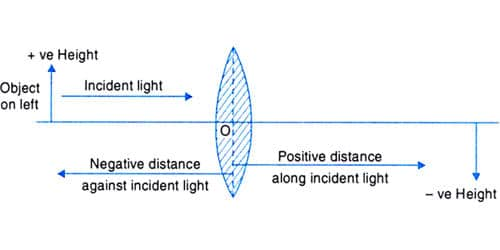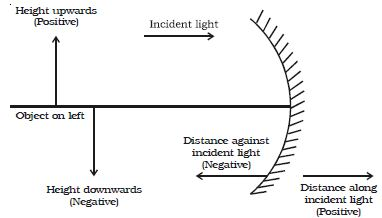Physics

# Explain Cartesian Sign ConventionAccording to the new Cartesian sign convention for the refraction of light through the concave and convex lens. The origin of the Cartesian coordinate system is at the center of the optical component. This sign convention is known as the Cartesian sign convention. The sign is taken as−(negative) from the pole of a spherical mirror towards the object along the principle axis.

In the case of a spherical mirror, all signs are taken from Pole of the spherical mirror, which is often called origin or origin point. This sign convention is known as New Cartesian Sign Convention. This means the sign is always taken as −(negative) in front of a spherical mirror. The following sign conventions are used by Cartesian:(1) All distances are measured from the pole of the mirror (in the case of the lens from the optic center). All the distances in a ray diagram of reflection by spherical mirrors are measured from the pole of the spherical mirror.

(2) The distances measured in the same direction as the incident light, are taken as positive. Acute angles are positive when produced by anticlockwise rotation from the optic axis, and negative when produced by clockwise rotation.

(3) The distances measured in the direction opposite to the direction of incident light are taken as negative.

(4) Heights measured perpendicular to the principal axis, in the upward direction are taken as positive. The heights measured upwards and perpendiculars to the principal axis of the mirror are taken as positive.

(5) Heights measured perpendicular to the principal axis, in the downward direction are taken as negative. The heights measured downwards and perpendiculars to the principal axis of the mirror are taken as negative.

(6) The size of the object is always taken as positive, but the image size is positive for erect image and negative for an inverted image.

(7) The magnification is positive for the erect (and virtual) image and negative for an inverted (and real) image.

When we deal with the reflection of light in spherical mirrors, we use a set of sign conventions called the New Cartesian Sign Convention. The pole (P) of the mirror is considered as the origin and the principal axis of the mirror is taken as the x-axis. The object is always placed to the left of the mirror. All distances parallel to the principal axis are measured from the pole of the mirror. All distances measured to the right of the origin are taken as positive while those measured to the left of the origin are taken as negative.